# Math in Focus Grade 3 Chapter 1 Practice 2 Answer Key Place value

Go through the Math in Focus Grade 3 Workbook Answer Key Chapter 1 Practice 2 Place value to finish your assignments.

## Math in Focus Grade 3 Chapter 1 Practice 2 Answer Key Place value

Read the numbers on each train. Fill in each blank.

Example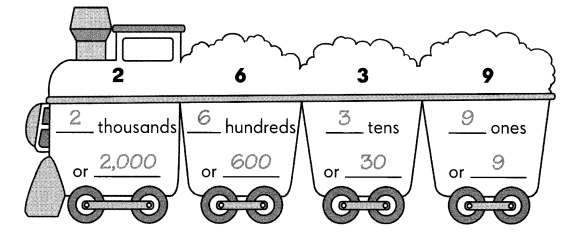Question 1.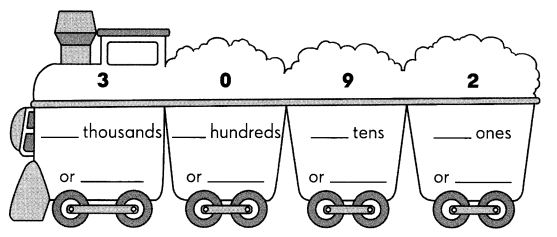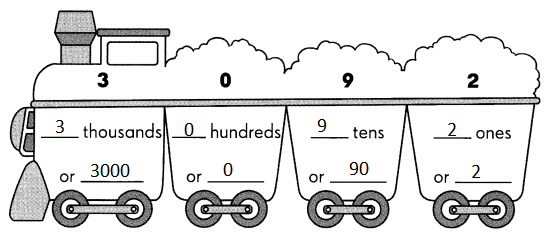Explanation:
The given standard form is converted to expanded form and word form

Question 2.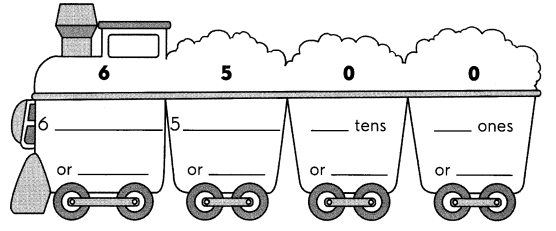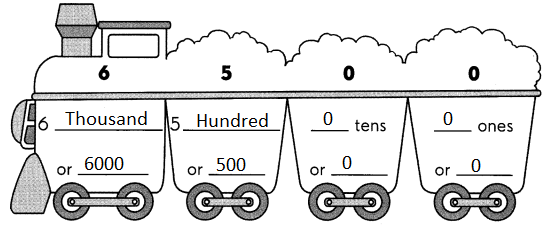Explanation:
The given standard form is converted to expanded form and word form

Write the value of each digit in the box.

Example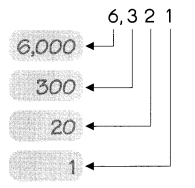Explanation:
Each digit has a fixed position called its place.
Each digit has a value depending on its place called the place value of the digit.

Question 3.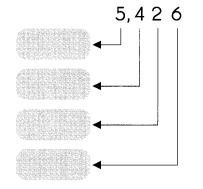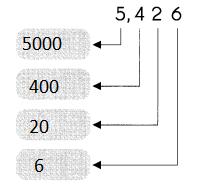Explanation:
Written the place value of every digit
Each digit has a fixed position called its place.
Each digit has a value depending on its place called the place value of the digit.

Question 4.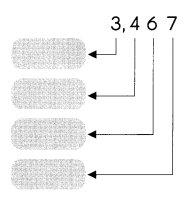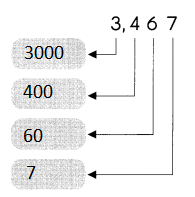Explanation:
Written the place value of every digit
Each digit has a fixed position called its place.
Each digit has a value depending on its place called the place value of the digit.

Question 5.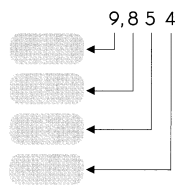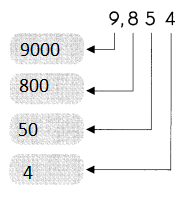Explanation:
Written the place value of every digit
Each digit has a fixed position called its place.
Each digit has a value depending on its place called the place value of the digit.

Question 6.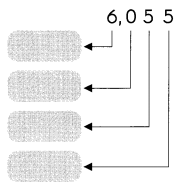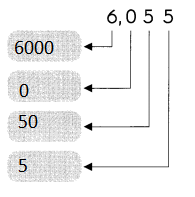Explanation:
Written the place value of every digit
Each digit has a fixed position called its place.
Each digit has a value depending on its place called the place value of the digit.

Question 7.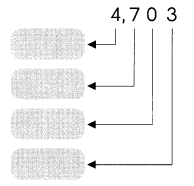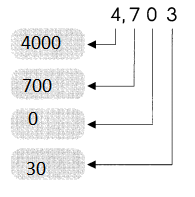Explanation:
Written the place value of every digit
Each digit has a fixed position called its place.
Each digit has a value depending on its place called the place value of the digit.

Question 8.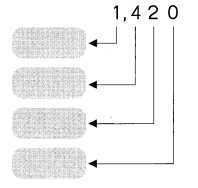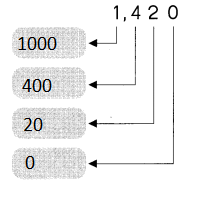Explanation:
Written the place value of every digit
Each digit has a fixed position called its place.
Each digit has a value depending on its place called the place value of the digit.

Question 9.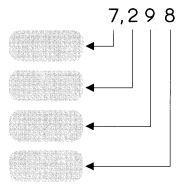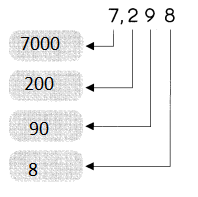Explanation:
Written the place value of every digit
Each digit has a fixed position called its place.
Each digit has a value depending on its place called the place value of the digit.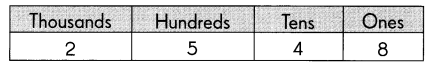Question 10.
In 2,548 the digit 4 is in the ___ place.
The value of the digit is ___.
In 2,548 the digit 4 is in the tens place.
The value of the digit is 40.
Explanation:
Each digit has a fixed position called its place.
Each digit has a value depending on its place called the place value of the digit.

Question 11.
In 2,548 the digit ___ is in the ones place.
The value of the digit is ___
In 2,548 the digit 8 is in the ones place.
The value of the digit is 8
Explanation:
Each digit has a fixed position called its place.
Each digit has a value depending on its place called the place value of the digit.

Question 12.
In 2,548 the value of the digit 2 is ___
It is in the __ place.
In 2,548 the value of the digit 2 is 2000
It is in the thousands place.
Explanation:
Each digit has a fixed position called its place.
Each digit has a value depending on its place called the place value of the digit.

Question 13.
In 2,548 the value of the digit ___ is 500.
It is in the ___ place.
In 2,548 the value of the digit 5 is 500.
It is in the hundreds place.
Explanation:
Each digit has a fixed position called its place.
Each digit has a value depending on its place called the place value of the digit.

Write each number in expanded form, standard form, and word form.

Example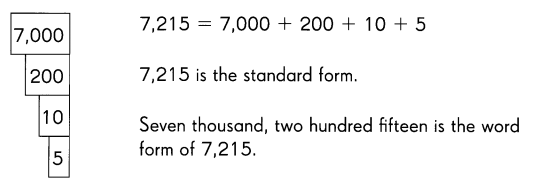Question 14.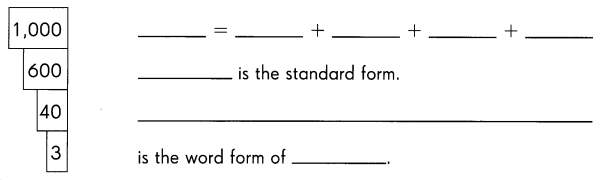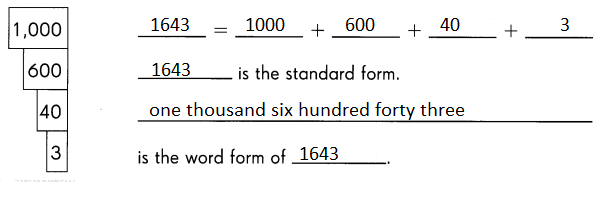Explanation:
The given tree is converted to expanded form and word form and standard form

Write each number in expanded form, standard form, and word form.

Question 15.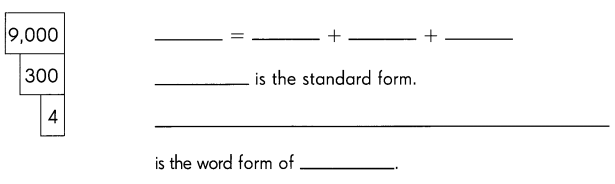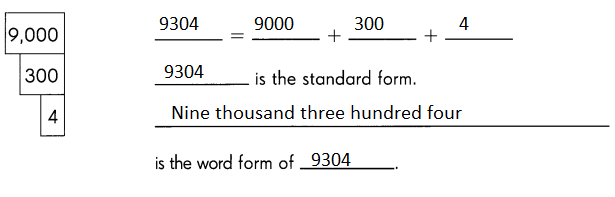Explanation:
The given tree is converted to expanded form and word form and standard form

Question 16.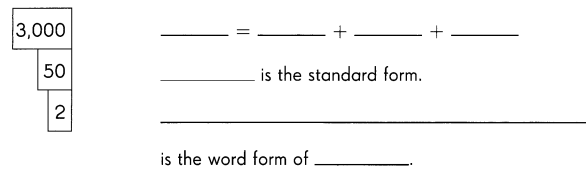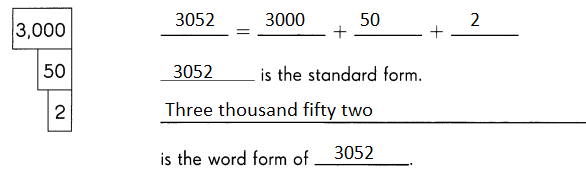Explanation:
The given tree is converted to expanded form and word form and standard form

Question 17.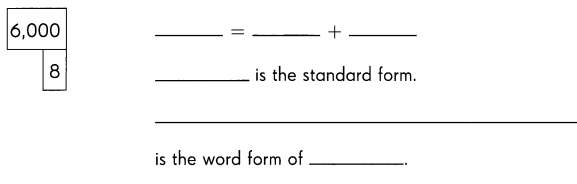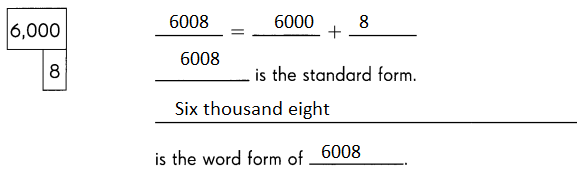Explanation:
The given tree is converted to expanded form and word form and standard form

Question 18.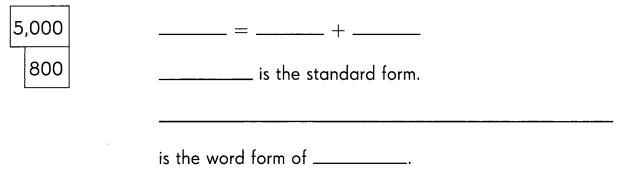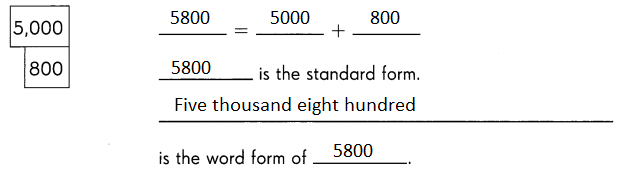Explanation:
The given tree is converted to expanded form and word form and standard form

Write each number shown.

Example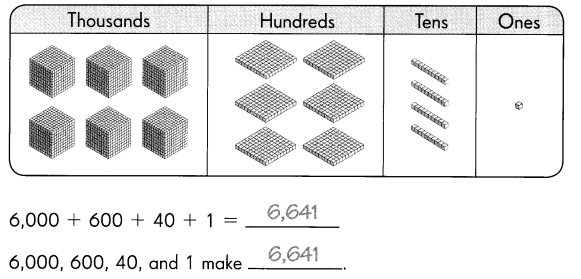Question 19.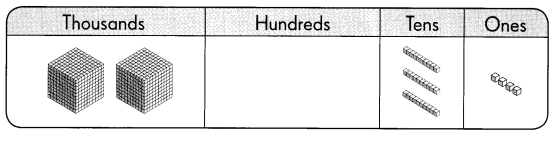2,000 + 30 + 4 = ___
2,000 + 30, and 4 make ___
2,000 + 30 + 4 = 2034
2,000 + 30, and 4 make 2034
Explanation:
Each cube value is thousand and square value is 100
line value is 10 and dot value is 1

Question 20.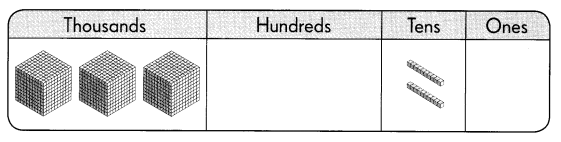3,000 + 20 = ___
3,000 + 20 make ___
3,000 + 20 = 3020
3,000 + 20 make 3020
Explanation:
Each cube value is thousand and square value is 100
line value is 10 and dot value is 1

Complete.

Question 21.
7,456 = 7,000 + __ + 50 + 6
7,456 = 7,000 + 400  + 50 + 6
Explanation:
Written the digits place of 4 is hundred
and value of its place is 400

Question 22.
6,391 = 6,000 + 300 + 90 + ___
6,391 = 6,000 + 300 + 90 + 1
Explanation:
Written the digits place of 1 is ones
and value of its place is 1

Question 23.
6,193 = 6,000 + 100 + ___ + 3
6,193 = 6,000 + 100 + 90 + 3
Explanation:
Written the digits place of 9 is tens
and value of its place is 90

Question 24.
6,107 = 6,000 + 100 + ___
6,107 = 6,000 + 100 + 7
Explanation:
Written the digits place of 7 is ones
and value of its place is 7

Question 25.
8,904 = __ + 900 + 4
8,904 = 8000 + 900 + 4
Explanation:
Written the digits place of 8 is Thousand
and value of its place is 8000

Question 26.
5,068 = ___ + 60 + 8
5,068 = 5000 + 60 + 8
Explanation:
Written the digits place of 5 is thousand
and value of its place is 5000

Question 27.
9,074 = 9,000 + ___ + 4